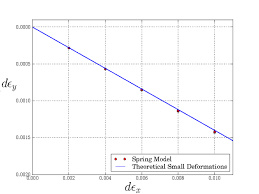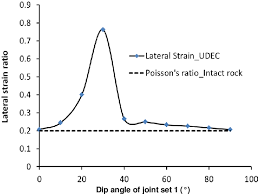## How to Calculate and Solve for Poisson’s Ratio | Rock MechanicsThe image above represents Poisson’s ratio.

To compute for Poisson’s ratio, two essential parameters are needed and these parameters are lateral strain (εland axial strain (εa).

The formula for calculating Poisson’s ratio:

v = εl / εa

Where:

v = Poisson’s Ratio
εl = Lateral Strain
εa = Axial Strain

Let’s solve an example;
Find the Poisson’s ratio when the lateral strain is 80 and the axial strain is 4.

This implies that;

εl = Lateral Strain = 80
εa = Axial Strain = 4

v = εl / εa
v = 80 / 4
v = 20

Therefore, the Poisson’s ratio is 20.

Calculating the Lateral Strain when the Poisson’s Ratio and Axial Strain is Given.

εl = v x εa

Where:

εl = Lateral Strain
v = Poisson’s Ratio
εa = Axial Strain

Let’s solve an example;
Find the lateral strain when the Poisson’s ratio is 40 and the axial strain is 9.

This implies that;

v = Poisson’s Ratio = 40
εa = Axial Strain = 9

εl = v x εa
εl = 40 x 9
εl = 360

Therefore, the lateral strain is 360.

## How to Calculate and Solve for Lateral Strain | Rock MechanicsThe image above represents lateral strain.

To compute for lateral strain, two essential parameters are needed and these parameters are change in diameter (Δd) and diameter (d).

The formula for calculating the lateral strain:

εl = Δd / d

Where:

εl = Lateral Strain
Δd = Change in Diameter
d = Diameter

Let’s solve an example;
Find the lateral strain when the change in diameter is 32 and the diameter is 14.

This implies that;

Δd = Change in Diameter = 32
d = Diameter = 14

εl = Δd / d
εl = 32 / 14
εl = 2.285

Therefore, the lateral strain is 2.285.

Calculating the Change in Diameter when the Lateral Strain and the Diameter is Given.

Δd = εl  x d

Where;

Δd = Change in Diameter
εl = Lateral Strain
d = Diameter

Let’s solve an example;
Find the change in diameter when the lateral strain is 40 and the diameter is 8.

This implies that;

εl = Lateral Strain = 40
d = Diameter = 8

Δd = εl  x d
Δd = 40 x 8
Δd = 320

Therefore, the change in diameter is 320.